Encoding two images (left and right) into two images (q and u) of the same size without data overhead.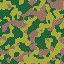(left)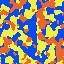(right)
Pseudo-Code:
```seed = hash(right);
r = Random(seed);
for (x,y) to (WIDTH, HEIGHT):
secret = r.nextInt(255);
q = left[x,y] + right[x,y] + secret;
u = left[x,y] - right[x,y] + secret;
```
Reconstruction can be done by subtraction q - u which results in left + right + secret - left + right - secret = 2 * right then divide it by two and the right image can be reconstructed. After that the hash of the reconstructed right image can be calculated and fed to the PRNG thus allowing left = q - right - secret. (Everything must be done using an odd modulus (e.g. 255))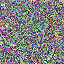(q)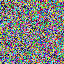(u)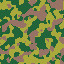(left reconstructed)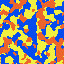(right reconstructed)
This method allows you to share two images to Alice and Bob while giving q to Alice and u to Bob. They will only be able to reconstruct the original images together. The good thing about this method is that you don't need to share a key or produce data overhead. However, I have no idea how secure it really is.